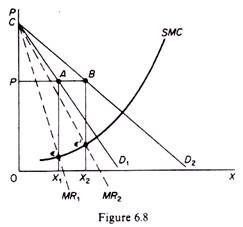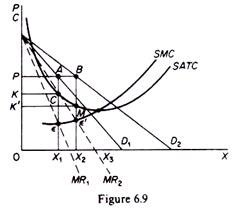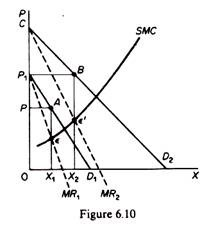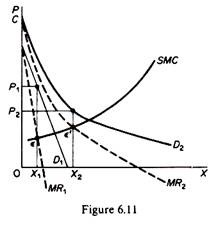# The Monopoly Model in Dynamic Situations (3 Situations)

We will examine the effects on the monopolist’s equilibrium of:

(a) A shift in the market demand,

(b) A change in costs,

(c) The imposition of a tax by the government.

A. shift in the market demand:

It is seen that an upward shift in the market demand resulted (in the short run) in a new market equilibrium with a higher price and a lower quantity. In a monopoly market this may not be so. An upward shift of the market demand (provided that the new demand does not intersect the initial one) will result in a new market equilibrium in which the quantity produced will be larger, but the price may increase, remain constant or decrease. Let us examine these possibilities.

In the new equilibrium the price may remain constant while the quantity supplied increases. This case is shown in figure 6.8. Assume that the new demand curve is D2, to the right of D1. The shift in D will lead to a shift of the MR curve (from MR1 to MR2). Given the marginal-cost curve of the monopolist, the new equilibrium position is e’ where the price is the same as before, but the quantity produced is larger (0X2 < 0X1).In this case the total revenue of the monopolist will increase (0PBX2 > 0PAX1). Furthermore his profits will be larger, because the monopolist’s SATC will be decreasing over the range between X1 and X2. This is so because the SMC curve cuts the SATC curve at its minimum point. Thus if at the initial equilibrium the monopolist was earning excess profits (equal to PKCA in figure 6.9), his SATC must have been above his SMC curve, and the SATC must have been declining, since it is impossible for it to start rising before it has been intersected by the SMC curve.

Assume that the point of inter­section of the SATC and the SMC curves occurs at the level of output X3. It is clear that at X2 the SATC is smaller than at the level X1. Hence the total excess profit of the monopolist increases if the price remains constant while his demand increases (PK’MB > PKCA in figure 6.9.)In the new equilibrium both the quantity and the price of the monopolist may be greater as compared to the initial equilibrium. This case is shown in figure 6.10. If the demand shifts to D2 (above D1) the new equilibrium is Ɛ’, at which the price as well as the quantity supplied by the monopolist are greater than at the original equilibrium e (clearly P2 > P1 and 0X2 > 0X1).In the new equilibrium the price may be lower than the initial while the quantity is larger. This is shown in figure 6.11.If demand shifts to D2 the quantity in the new equilibrium will be larger (0X2 > 0X1) and it will be sold at a lower price (P2 < P1). It should be clear that the effects of the shift in demand depend on the extent of the shift and on the price elasticity of demand. When demand shifts its elasticity is changing at any one price. If the new demand curve lies above the original and has a substantially greater elasticity (at the original price level), it may be profitable for the monopolist not only to increase his output, but also to sell it at a lower price.

B. An increase in the costs of the monopolist:

The analysis of the effects of a change in costs of the monopolist is the same as in the case of pure competition.

An increase in fixed costs:

If the fixed costs of the monopolist increase, his short-run equilibrium will not be affected, since his demand is given and his SMC is not affected by changes in fixed costs. This is the same result as in pure competition. In the long run also the equilibrium of the monopolist will not be affected so long as the increase in fixed costs is covered by excess profits. If, however, the increase in fixed costs is so substantial as to make the SATC shift above the market demand, the monopolist will close down, since at all levels of output he will not be able to cover his total costs.

An increase in the variable costs:

If the variable costs increase, the MC curve of the monopolist will shift upwards to the left with the consequence of a reduction in the output and an increase in the price. The same direction of changes are obtained in a pure competition model. The difference between the two markets is that the changes in price and market output will be greater in pure competition. This is due to the fact that the monopolist equates his MC to his MR (and not to price) while the firms in pure competition equate MC to price.

The slope of the MR of the monopolist is steeper than the slope of his AR curve, while in pure competition the two curves are the same (MR = AR = P). Consequently the same vertical (upwards) shift of the MC in the two markets results in a smaller decrease in the quantity of the monopolist and hence to a smaller increase in the price as compared with the purely competitive market.

This is shown in figures 6.12 and 6.13. In both cases it is assumed that the market demand is the same and the increase in the variable costs shifts the MC curves of the firms identically. Yet the increase in price and the decline in the quantity are more accentuated in the purely competitive market. This leads to the conclusion that price fluctuations and employment changes caused by changes in factor prices will tend to be wider in pure competition than in monopoly (given the market demand).

C. imposition of a tax:

We will examine the effects on the equilibrium of the monopolist of:

(a) A lump-sum tax,

(b) A profits tax,

(c) A specific sales tax.

Imposition of a lump-sum tax (per period):

In the case of a monopolist we need not distin­guish between the short run and the long run as we did in the purely competitive market because in general the monopolist realizes some excess profits both in the short run and in the long run. Under these conditions the imposition of a lump tax will reduce the excess profits of the monopolist because it will increase his total fixed cost. However, the MC curve of the monopolist will not be affected, and hence the equilibrium in the monopoly market will remain the same even in the long run (provided that the lump-sum tax does not exceed the supernormal profits of the monopolist).

Imposition of a profits tax:

The effects of taxes on the monopoly profits on the equilibrium of the monopolist are the same as in the case of the lump-sum tax: the profits tax reduces the abnormal (monopoly) profits, but the equilibrium in the market is not affected, so long as the profits tax does not bite into the normal profits of the monopolist, since in this event the monopolist will not be covering his total costs (inclusive of his normal profit) and will close down.

Imposition of a specific sales tax:

The effects of a specific tax on the output of the monop­olist are broadly the same with those in a purely competitive market. The imposition of the specific tax will shift the MC curve of the monopolist upwards, which will result in a change of his equilibrium; in the new equilibrium position (Ɛ’) the price will be higher and the quantity smaller as compared with the initial equilibrium. This is the same qualitative prediction with the model of pure competition.

The change in the price of the monopolist may be smaller, equal or greater than the specific tax, as in the case of pure competition. However, in the monopoly market we do not distinguish between the short run and the long run, since the conditions of equilibrium are the same in both periods.

Firstly:

If the MC of the monopolist has a positive slope, the increase in the price will be smaller than the specific tax, as in the case of pure competition. The monopolist will pass to the consumer part of the specific tax (in figure 6.14 ΔP < tax).

Secondly:

If the MC of the monopolist is horizontal, the monopolist will raise the price, but not by the full amount of the tax, as is the case in pure competition. Even when his MC curve is infinitely elastic, the monopolist will bear some amount of the specific tax (in figure 6.15 ∆P < tax).

Thirdly:

The examination of the conditions under which the monopolist can pass the total tax to the consumer by charging a suitably higher price, or can raise the-price more than the amount of the tax, is too complicated to be attempted here. The interested reader is referred to textbooks on public finance and public policy.